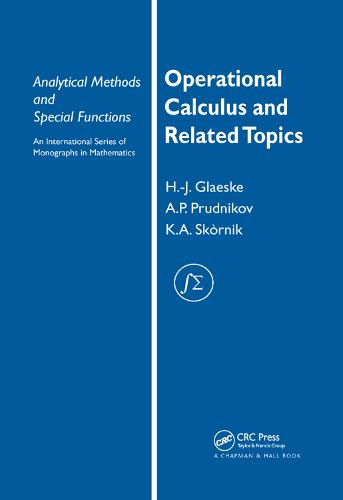•# Operational Calculus and Related Topics - Analytical Methods and Special Functions (Paperback)

(author), (author)
£54.99
Paperback 424 Pages / Published: 11/09/2019
• We can order this

Usually dispatched within 3 weeks

Even though the theories of operational calculus and integral transforms are centuries old, these topics are constantly developing, due to their use in the fields of mathematics, physics, and electrical and radio engineering. Operational Calculus and Related Topics highlights the classical methods and applications as well as the recent advances in the field.

Combining the best features of a textbook and a monograph, this volume presents an introduction to operational calculus, integral transforms, and generalized functions, the backbones of pure and applied mathematics. The text examines both the analytical and algebraic aspects of operational calculus and includes a comprehensive survey of classical results while stressing new developments in the field. Among the historical methods considered are Oliver Heaviside's algebraic operational calculus and Paul Dirac's delta function. Other discussions deal with the conditions for the existence of integral transforms, Jan Mikusinski's theory of convolution quotients, operator functions, and the sequential approach to the theory of generalized functions.

Benefits...

* Discusses theory and applications of integral transforms

* Gives inversion, complex-inversion, and Dirac's delta distribution formulas, among others

* Offers a short survey of actual results of finite integral transforms, in particular convolution theorems

Because Operational Calculus and Related Topics provides examples and illustrates the applications to various disciplines, it is an ideal reference for mathematicians, physicists, scientists, engineers, and students.

Publisher: Taylor & Francis Ltd
ISBN: 9780367390495
Number of pages: 424
Weight: 776 g
Dimensions: 254 x 178 mm